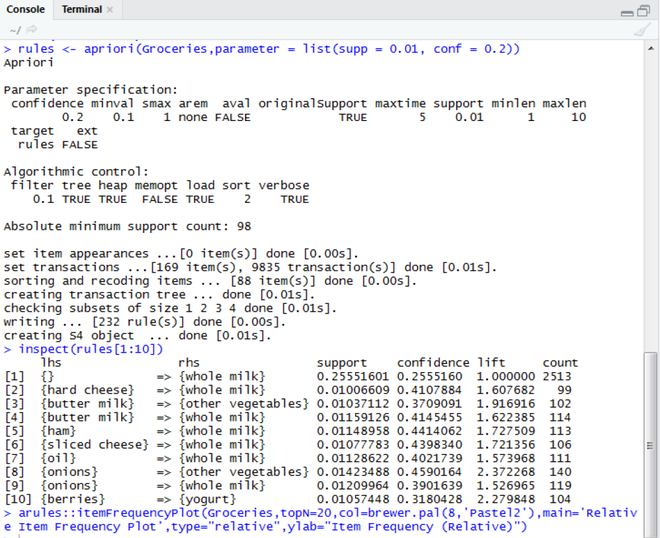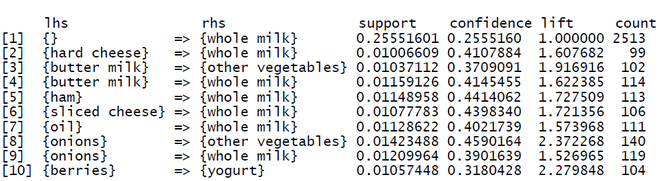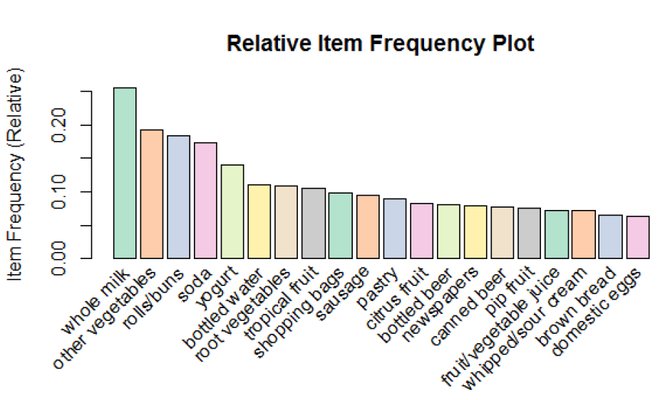# Apriori Algorithm in R Programming

Apriori algorithm is used for finding frequent itemsets in a dataset for association rule mining. It is called Apriori because it uses prior knowledge of frequent itemset properties. We apply an iterative approach or level-wise search where k-frequent itemsets are used to find k+1 itemsets. To improve the efficiency of the level-wise generation of frequent itemsets an important property is used called Apriori property which helps by reducing the search space. It’s very easy to implement this algorithm using the R programming language.

Apriori Property: All non-empty subsets of a frequent itemset must be frequent. Apriori assumes that all subsets of a frequent itemset must be frequent (Apriori property). If an itemset is infrequent, all its supersets will be infrequent.

Essentially, the Apriori algorithm takes each part of a larger data set and contrasts it with other sets in some ordered way. The resulting scores are used to generate sets that are classed as frequent appearances in a larger database for aggregated data collection. In a practical sense, one can get a better idea of the algorithm by looking at applications such as a Market Basket Tool that helps with figuring out which items are purchased together in a market basket, or a financial analysis tool that helps to show how various stocks trend together. The Apriori algorithm may be used in conjunction with other algorithms to effectively sort and contrast data to show a much better picture of how complex systems reflect patterns and trends.

### ImportantTerminologies

• Support: Support is an indication of how frequently the itemset appears in the dataset. It is the count of records containing an item ‘x’ divided by the total number of records in the database.
• Confidence: Confidence is a measure of times such that if an item ‘x’ is bought, then item ‘y’ is also bought together. It is the support count of (x U y) divided by the support count of ‘x’.
• Lift: Lift is the ratio of the observed support to that which is expected if ‘x’ and ‘y’ were independent. It is the support count of (x U y) divided by the product of individual support counts of ‘x’ and ‘y’.

### Algorithm

1. Read each item in the transaction.
2. Calculate the support of every item.
3. If support is less than minimum support, discard the item. Else, insert it into frequent itemset.
4. Calculate confidence for each non- empty subset.
5. If confidence is less than minimum confidence, discard the subset. Else, it into strong rules.

### Apriori Algorithm Implementation in R

RStudio provides popular open source and enterprise-ready professional software for the R statistical computing environment. R is a language that is developed to support statistical calculations and graphical computing/ visualizations. It has an in-built library function called arules which implements the Apriori algorithm for Market Basket Analysis and computes the strong rules through Association Rule Mining, once we specify the minimum support and minimum confidence, according to our needs. Given below are the required code and corresponding output for the Apriori algorithm. The Groceries dataset has been used for the same, which is available in the default database of R. It contains 9,835 transactions/ records, each having ‘n’ number of items that were bought together from the grocery store.

Example:

arules’ package provides the infrastructure for representing, manipulating, and analyzing transaction data and patterns.

`library(arules)`

arulesviz’ package is used for visualizing Association Rules and Frequent Itemsets. It extends the package ‘arules’ with various visualization techniques for association rules and itemsets. The package also includes several interactive visualizations for rule exploration.

`library(arulesViz)`

RColorBrewer‘ is a ColorBrewer Palette which provides color schemes for maps and other graphics.

`library(RColorBrewer)`

Step 2: Import the dataset

Groceries‘ dataset is predefined in the R package. It is a set of  9835 records/ transactions, each having ‘n’ number of items, which were bought together from the grocery store.

`data("Groceries")`

Step 3: Applying apriori() function

apriori()‘ function is in-built in R to mine frequent itemsets and association rules using the Apriori algorithm. Here, ‘Groceries’ is the transaction data. ‘parameter’ is a named list that specifies the minimum support and confidence for finding the association rules. The default behavior is to mine the rules with minimum support of 0.1 and 0.8 as the minimum confidence. Here, we have specified the minimum support to be 0.01 and the minimum confidence to be 0.2.

`rules <- apriori(Groceries, parameter = list(supp = 0.01, conf = 0.2))`

Step 4: Applying inspect() function

inspect() function prints the internal representation of an R object or the result of an expression. Here, it displays the first 10 strong association rules.

`inspect(rules[1:10])`

Step 5: Applying itemFrequencyPlot() function

itemFrequencyPlot() creates a bar plot for item frequencies/ support. It creates an item frequency bar plot for inspecting the distribution of objects based on the transactions. The items are plotted ordered by descending support. Here, ‘topN=20’ means that 20 items with the highest item frequency/ lift will be plotted.

```arules::itemFrequencyPlot(Groceries, topN = 20,
col = brewer.pal(8, 'Pastel2'),
main = 'Relative Item Frequency Plot',
type = "relative",
ylab = "Item Frequency (Relative)")```

The complete R code is given below.

## R

 `# Loading Libraries` `library``(arules)` `library``(arulesViz)` `library``(RColorBrewer)`   `# import dataset` `data``(``"Groceries"``)`   `# using apriori() function` `rules <- ``apriori``(Groceries, ` `                 ``parameter = ``list``(supp = 0.01, conf = 0.2))`   `# using inspect() function` `inspect``(rules[1:10])`   `# using itemFrequencyPlot() function` `arules::``itemFrequencyPlot``(Groceries, topN = 20, ` `                          ``col = ``brewer.pal``(8, ``'Pastel2'``),` `                          ``main = ``'Relative Item Frequency Plot'``,` `                          ``type = ``"relative"``,` `                          ``ylab = ``"Item Frequency (Relative)"``)`

Output:Strong Rules:

Strong Rules obtained after applying the Apriori Algorithm is as followsAfter running the above code for the Apriori algorithm, we can see the following output, specifying the first 10 strongest Association rules, based on the support (minimum support of 0.01), confidence (minimum confidence of 0.2), and lift, along with mentioning the count of times the products occur together in the transactions.

Visualization:

Box Plot of the Top 20 Items having the Highest Item Frequency (Relative) using Lift as a Parameter### Conclusion

We have used the ‘Groceries’ dataset which has about 9835 transactions that include ‘n’ number of items that were bought together from the store. On running the Apriori algorithm over the dataset with a minimum support value of 0.01 and minimum confidence of 0.2, we have filtered out the strong association rules in the transaction. We have listed the first 10 transactions above, along with the box plot of the top 20 items having the highest relative item frequency. Some association rules that we can conclude from this program are:

• If hard cheese is bought, then whole milk is also bought.
• If buttermilk is bought, then whole milk is also bought with it.
• If buttermilk is bought, then other vegetables are also bought together.
• Also, whole milk has high support as well as a confidence value.

Hence, it will be profitable to put ‘whole milk’ in a visible and reachable shelf as it is one of the most frequently bought items. Also, near the shelf where ‘buttermilk’ is put, there should be shelves for ‘whole milk’ and ‘other vegetables’ as their confidence value is quite high. So there is a higher probability of buying them along with buttermilk. Thus, with similar actions, we can aim at increasing the sales and profits of the grocery store by analyzing users’ shopping patterns.

Whether you're preparing for your first job interview or aiming to upskill in this ever-evolving tech landscape, GeeksforGeeks Courses are your key to success. We provide top-quality content at affordable prices, all geared towards accelerating your growth in a time-bound manner. Join the millions we've already empowered, and we're here to do the same for you. Don't miss out - check it out now!

Previous
Next